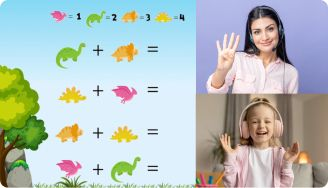# Comparing Fractions With Unlike Denominators Worksheets

Comparing fractions with unlike denominators is certainly no walk in the park, even for most math geniuses. Fortunately, with comparing fractions with unlike denominators worksheets, your kid can learn how to compare this type of fractions and perform similar operations.

Leave your email and we will send you worksheets

worksheets sent successfully

## About a Comparing Fractions with Unlike Denominators Worksheet

Most 3rd graders understand what fractions as well as numerators and denominators are. However, when it comes to working with unlike denominators, they run into a brick wall. This is mainly because comparing such fractions is a multi-step process. First, kids need to convert the fractions to like denominators before they begin the comparison.

This is where our comparing unlike fractions worksheet comes in. For starters, the worksheet teaches young learners about the importance of common denominators when comparing multiple fractions. Kids will find tons of questions that will test their knowledge and help them work with unlike denominators. Over time, they’ll become gurus at fractions.

1:1 Math Lessons

Want to raise a genius?
Start learning Math with Brighterly## Benefits of Using a Comparing Fractions Unlike Denominators Worksheet

Wondering why you need to try out the comparing fractions with unlike denominators worksheet pdf? Here’s why:

For starters, it helps to build students’ basic knowledge of fractions. They learn more about fractions, numerators, and denominators. They will also learn how to identify unlikely denominators. But that’s not where it ends. The worksheet goes further to teach kids how to decipher unlike denominators, simplify fractions, and work with said denominators.

Leave your email and we will send you worksheets

worksheets sent successfully### Comparing Fractions With Unlike Denominators Worksheets PDF

View worksheet

Comparing Fractions With Unlike Denominators Worksheet### Comparing Fractions With Unlike Denominators Worksheets PDF

View worksheet

Comparing Fractions With Different Denominators Worksheet### Comparing Fractions With Unlike Denominators Worksheets PDF

View worksheet

Comparing Unlike Fractions Worksheet### Comparing Fractions With Unlike Denominators Worksheets PDF

View worksheet

Comparing Fractions Unlike Denominators Worksheet

With a wide range of fun exercises, a child comes to see these fractions as an exciting challenge rather than a huge obstacle they need to overcome.

### More Fractions Worksheets

Book 1 to 1 Math Lesson• Specify your child’s math level
• Get practice worksheets for self-paced learning
• Your teacher sets up a personalized math learning plan for your childBook 1 to 1 Math Lesson